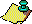Contents Next: A heuristic argument. Up: No Title Previous: Introduction

# The 3x+1 problem.

The known results on the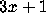problem are most elegantly expressed in terms of iterations of the function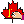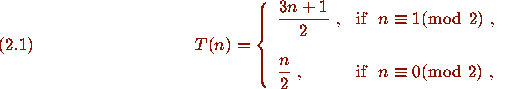One way to think of theproblem involves a directed graph whose vertices are the positive integers and that has directed edges from n to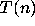. I call this graph the Collatz graph ofin honor of L. Collatz . A portion of the Collatz graph of T(n) is pictured in the Figure below.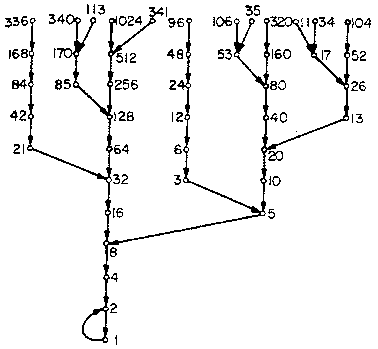(Follow this link to see the definition of the Collatz function and to experiment with Gaston Gonnet's Fast Maple Code for computing the stopping time function for the Collatz function!) A directed graph is said to be weakly connected if it is connected when viewed as an undirected graph, i.e., for any two vertices there is a path of edges joining them, ignoring the directions on the edges. TheConjecture can be formulated in terms of the Collatz graph as follows.

### 3x+1 CONJECTURE (First form).

The Collatz graph ofon the positive integers is weakly connected.

We call the sequence of iterates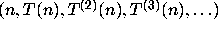the trajectory of n. There are three possible behaviors for such trajectories when n > 0.

(i). Convergent trajectory. Some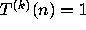.
(ii). Non-trivial cyclic trajectory. The sequence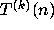eventually becomes periodic and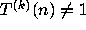for any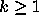.
(iii). Divergent trajectory.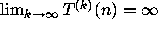.

TheConjecture asserts that all trajectories of positive n are convergent. It is certainly true for n > 1 thatcannot occur without some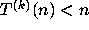occurring. Call the least positive k for whichthe stopping time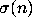of n, and set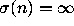if no k occurs with. Also call the least positive k for whichthe total stopping time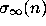of n, and set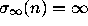if no such k occurs. We may restate theConjecture in terms of the stopping time as follows.

### 3x+1 CONJECTURE (Second form).

Every integer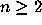has a finite stopping time.

The appeal of theproblem lies in the irregular behavior of the successive iterates. One can measure this behavior using the stopping time, the total stopping time, and the expansion factor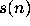defined by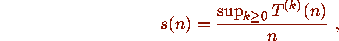if n has a bounded trajectory and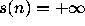if n has a divergent trajectory. For example n = 27 requires 70 iterations to arrive at the value 1 and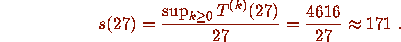Table 1 illustrates the concepts defined so far by giving data on the iteratesfor selected values of n.

TABLE 1. Behavior of iterates.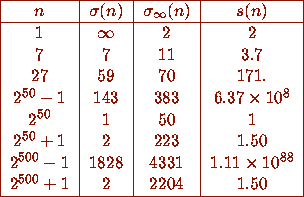TheConjecture has been numerically checked for a large range of values of n. It is an interesting problem to find efficient algorithms to test the conjecture on a computer. The current record for verifying theConjecture seems to be held by Nabuo Yoneda at the University of Tokyo, who has reportedly checked it for all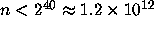. In several places the statement appears that A. S. Fraenkel has checked that all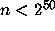have a finite total stopping time; this statement is erroneous .Contents Next: A heuristic argument. Up: No Title Previous: Introduction